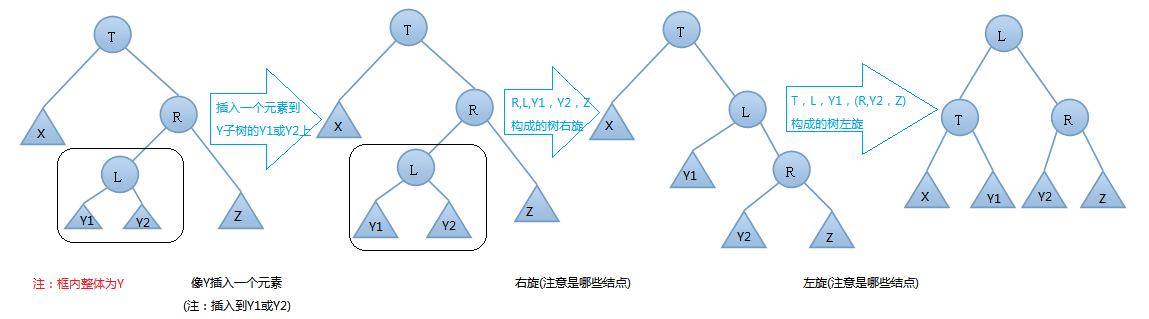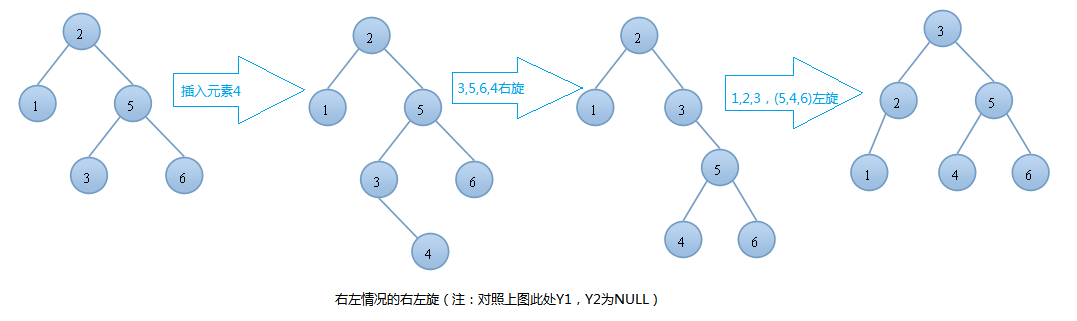# 圖解AVL樹數據結構輸入與輸出及實現示例

## AVL樹(平衡二叉樹)：

AVL樹本質上是一顆二叉查找樹，但是它又具有以下特點：它是一棵空樹或它的左右兩個子樹的高度差的絕對值不超過1，並且左右兩個子樹都是一棵平衡二叉樹。在AVL樹中任何節點的兩個子樹的高度最大差別為一，所以它也被稱為平衡二叉樹。下面是平衡二叉樹和非平衡二叉樹對比的例圖：## AVL樹的作用：## AVL樹的基本操作：

AVL樹的操作基本和二叉查找樹一樣，這裡我們關註的是兩個變化很大的操作：插入和刪除！

### AVL樹的插入，單旋轉的第一種情況—右旋：T向右旋轉成為L的右結點，同時，Y放到T的左孩子上。這樣即可得到一顆新的AVL樹，旋轉過程圖如下：### AVL樹的插入，單旋轉的第二種情況—左旋：T向右旋轉成為R的左結點，同時，Y放到T的左孩子上。這樣即可得到一顆新的AVL樹，旋轉過程圖如下：### AVL樹的插入，雙旋轉的第一種情況—左右(先左後右)旋：### AVL樹的插入，雙旋轉的第二種情況—右左(先右後左)旋：## AVL樹的插入代碼實現:(僅供參考)

```#include <iostream>
using namespace std;
#define DataType int
/*
定義AVL樹的結構體，鏈式
*/
typedef struct AvlNode{
DataType    data;
AvlNode    * m_pLeft;
AvlNode    * m_pRight;
int height;
}*AvlTree,*Position,AvlNode;
//求兩個數的最大值
int Max(int a,int b)
{
return a>b?a:b;
}
//求樹的高度
int Height( AvlTree T)
{
if(NULL == T)
return -1;
else
return T->height;
}
//單旋轉右旋
AvlTree singleRotateWithRight(AvlTree T)
{
AvlTree L = T->m_pLeft;
T->m_pLeft = L->m_pRight;
L->m_pRight = T;
T->height = Max( Height(T->m_pLeft),Height(T->m_pRight) ) + 1;
L->height = Max( Height(L->m_pLeft),Height(L->m_pRight) ) + 1;
return L;    //此時L成為根節點瞭（可參考AVL的插入的左左情況的右旋圖）
}
//單旋轉左旋
AvlTree singleRotateWithLeft(AvlTree T)
{
AvlTree R = T->m_pRight;
T->m_pRight = R->m_pLeft;
R->m_pLeft = T;
T->height = Max( Height(T->m_pLeft),Height(T->m_pRight) ) + 1;
R->height = Max( Height(R->m_pLeft),Height(R->m_pRight) ) + 1;
return R;    //此時R成為根節點瞭（可參考AVL的插入的左左情況的左旋圖）
}
//雙旋轉，先左後右
AvlTree doubleRotateWithLeft(AvlTree T)        //先左後右
{
T->m_pLeft = singleRotateWithLeft(T->m_pLeft);
return singleRotateWithRight(T);
}
//雙旋轉，先右後左
AvlTree doubleRotateWithRight(AvlTree T)    //先右後左
{
T->m_pRight = singleRotateWithRight(T->m_pRight);
return singleRotateWithLeft(T);
}
AvlTree AvlTreeInsert(AvlTree T, DataType x)
{
if(T == NULL)    //如果樹為空
{
T = (AvlNode *)malloc(sizeof(struct AvlNode));
if(T)
{
T->data = x;
T->m_pLeft    = NULL;
T->m_pRight = NULL;
T->height = 0;
}
else
{
cout << "空間不夠" << endl;
exit(0);
}
}
else if( x < T->data)        //如果插入到T結點的左子樹上
{
T->m_pLeft = AvlTreeInsert(T->m_pLeft,x);    //先插入，後旋轉
if(Height(T->m_pLeft) - Height(T->m_pRight) == 2) //隻有可能是這個
{
if(x < T->m_pLeft->data)        //左左情況，隻需要右旋轉
{
T = singleRotateWithRight( T );
}
else                            //左右情況，雙旋轉,先左
{
T = doubleRotateWithLeft( T );
}
}
}
else if( x > T->data )
{
T->m_pRight = AvlTreeInsert(T->m_pRight,x);
if(Height(T->m_pRight) - Height(T->m_pLeft) == 2)
{
if(x > T->m_pRight->data)        //右右情況，進行左旋
{
T = singleRotateWithLeft( T );
}
else                            //左右情況，雙旋轉,先右
{
T = doubleRotateWithRight( T );
}
}
}
//如果這個數已經存在，那麼不進行插入
T->height = Max(Height(T->m_pLeft),Height(T->m_pRight)) + 1;
return T;
}
//遞歸實現中序遍歷
void inOrderVisitUseRecur(const AvlTree pCurrent)
{
if(pCurrent)
{
inOrderVisitUseRecur(pCurrent->m_pLeft);
cout << pCurrent->data << " ";
if(pCurrent->m_pLeft)
cout << " leftChild: "<<pCurrent->m_pLeft->data;
else
cout << " leftChild: "<<"NULL" ;
if(pCurrent->m_pRight)
cout << " rightChild: "<<pCurrent->m_pRight->data;
else
cout << " rightChild: "<< "NULL";
cout << endl;
inOrderVisitUseRecur(pCurrent->m_pRight);
}
}
int main()
{
AvlTree root = NULL;
root = AvlTreeInsert(root,1);
root = AvlTreeInsert(root,2);
root = AvlTreeInsert(root,3);
root = AvlTreeInsert(root,4);
root = AvlTreeInsert(root,5);
root = AvlTreeInsert(root,6);
root = AvlTreeInsert(root,7);
root = AvlTreeInsert(root,8);
root = AvlTreeInsert(root,9);
root = AvlTreeInsert(root,10);
root = AvlTreeInsert(root,11);
root = AvlTreeInsert(root,12);
root = AvlTreeInsert(root,13);
root = AvlTreeInsert(root,14);
root = AvlTreeInsert(root,15);
inOrderVisitUseRecur(root);
return 0;
}```